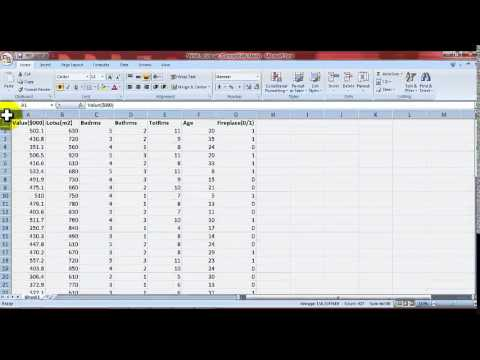# Microsoft Excel data analysis tool for statistics mean, median, hypothesis, regression

This video covers a few topics using the data analysis tool.

After this video you should be able to:

a) Find and use data analysis on excel to calculate statistics

b) Calculate the mean, median, mode, standard deviation, range and coefficient variation on a variable set of data in excel.

c) Conduct a confidence interval in excel.

d) Complete a T-test in excel to help complete a hypothesis test.

e) Conduct a linear regression analysis output from excel and create a scatter diagram.

229 – 1397284947 – 80804

data analysis excel